Refer to our Texas Go Math Grade 2 Answer Key Pdf to score good marks in the exams. Test yourself by practicing the problems from Texas Go Math Grade 2 Unit 1 Assessment Answer Key.

Vocabulary

Use a word in the box to complete the sentence.
thousand
halves
hundred

Question 1.
10 tens is the same as 1 ___________. (p.26)
Explanation:
10 Tens  = 1 hundred

Question 2.
There are 2 ______________ in one whole. (p.116)
There are 2 halves in one whole.
Explanation:
2 x half = 1

Concepts and Skills

Draw and label points for 315, 398, and 407 on the number line. (TEKS 2.2.E)

Question 3.Explanation:
Based on ascending order of numbers, labeled the points on line.

Compare the numbers. Write >, <, or =.

Question 4.Explanation:
362 is greater than 358

Question 5.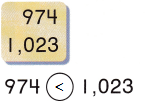Explanation:
974 is less than  1023

Write the sum. (TEKS 2.4.B)

Question 6.Explanation:
The sum of 56 and 35 is 91

Question 7.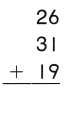Explanation:
The sum of 26, 31 and 19 is 76

Question 8.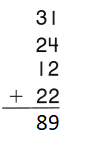Explanation:
The sum of 31, 24, 12 and 22 is 89

Fill in the bubble for the correct answer choice.

Question 9.
Mr. Garcia cut some sandwiches into parts that are fourths. How many fourths should he put on a plate to have one whole sandwich? (TEKS 2.3.C)
(A) 4
(B) 2
(C) 8
Explanation:
4 x fourths = 1

Question 10.
Mr. Grant has 357 sheets of paper. Ms. Kim has more sheets of paper. Which of these could be the number of sheets of paper that Ms. Kim has? (TEKS 2.2.C)
(A) 348
(B) 299
(C) 360
Explanation:
299 and 348 are less than 357
360 is greater than 357

Question 11.
Billy used these blocks to model a number. What number can be shown as 11 hundreds, 5 tens, and 3 ones? (TEKS 2.2.A)(A) 1,153
(B) 1,108
(C) 1,603
Explanation:
11 hundreds,  5 tens, and 3 ones = 1100 + 50 + 3 = 1153

Question 12.
Mrs. Philips has a box of 10 erasers and a box of 7 erasers. She gives 8 erasers to her students. How many erasers does Mrs. Philips have now? (TEKS 2.4.A)(A) 9
(B) 8
(C) 25
Explanation:
Mrs. Philips has a box of 10 erasers and a box of 7 erasers.
So total she has 17 erasers since 10 + 7 = 17
She gives 8 erasers to her students
9 erasers Mrs. Philips have now. Since
17 – 8 = 9

Fill in the bubble for the correct answer choice.

Question 13.
Which shape is divided into fourths? (TEKS 2.3.D)Explanation:
Rectangle equally divided into fourths

Question 14.
Tyler used these blocks to model a number. What number can be shown as 4 hundreds, 12 tens, and 7 ones? (TEKS 2.2.A)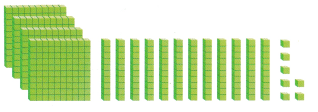(A) 507
(B) 427
(C) 527
Explanation:
4 hundreds, 12 tens, and 7 ones
= 400 + 120 + 7
= 520 + 7
=527

Question 15.
Which number belongs at the point on this number line? (TEKS 2.2.F)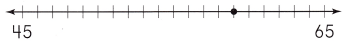(A) 55
(B) 60
(C) 59
C
Explanation:Question 16.
The shading shows how many pieces of pizza have not been eaten yet. Which describes how much pizza there is? (TEKS 2.3.C)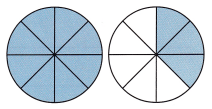(A) one and 3 eighths
(B) one and 1 eighth
(C) one and 8 eighths
Explanation:
one and 3 eighths describes that how much pizza there is

Fill in the bubble for the correct answer choice.

Question 17.
Keira has 2 packs of stickers. There are 35 stickers in the first pack. There are 28 stickers in the second pack. How many stickers does Keira have? (TEKS 2.4.B)(A) 65
(B) 63
(C) 73
B
Explanation:
Keira has 2 packs of stickers.
There are 35 stickers in the first pack.
There are 28 stickers in the second pack.
35 + 28 = 63

Question 18.
Megan has two posters that are the same size. Draw to show one poster divided into fourths. Draw to show the other poster divided into eighths. (TEKS 2.3.A)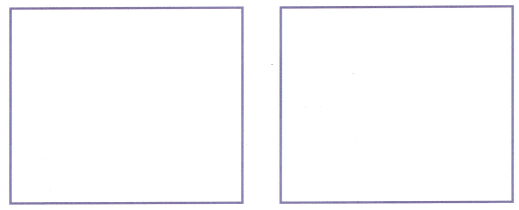Suppose Megan covers 3 of the equal parts on each poster with stickers. Which poster will have a greater amount that is covered with stickers? Explain. (TEKS 2.3.B)